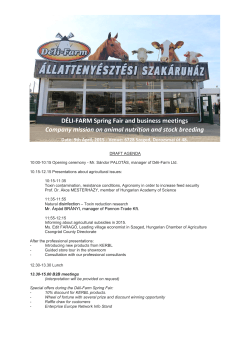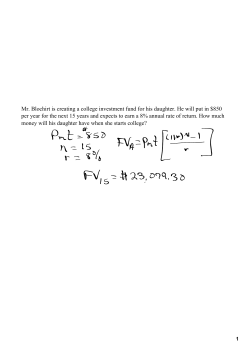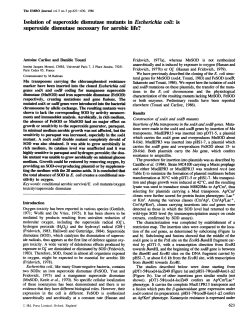# Homework: Multi-arm Bandit Framework Deadline: March 3

```EC: Reinforcement Learning
Lecture: 1
Homework: Multi-arm Bandit Framework
Lecturer: Alessandro Lazaric
http://researchers.lille.inria.fr/∼lazaric/Webpage/Teaching.html
1
The Soda Vending Machine Problem
Description of the Problem
The soda vending machine offers two kinds of soda: energy drink (E) or sugar free drink (N). E and N are
sold at the same price of 1A
Cand at the beginning the machine contains the same amount of sodas (50 cans
for each type). On a normal day, E has a slightly higher probability to be chosen, while on exam days E has
a much higher probability to be chosen. Whenever one of the sodas is finished, the machine gets restock.
Since refilling the machine is an expensive operation, the objective is to maximize the amount of money
gained between two restocks. In order to achieve this objective, the machine can automatically decide to
discount one of the two sodas in order to incentive the consumption of a soda.
Implementation of the Environment
The environment together with the preferences model and the evolution between normal and exam days is
Discount strategy
Given a discount strategy, the performance is evaluated as the average reward collected over R restocks (see
test simulator.m).
The objective of the homework is to
• Define a parameterized discount strategy. You can take inspiration from the soda strategy param.m.
An example of parameters could be the amount of discount, the amount of sodas available before
starting the discount, the amount of money already collected.
• Fix a value for the parameters at hand and compare its performance with the two baselines soda strategy discount.m
and soda strategy nodiscount.m.
• Implement a bandit strategy such that each round r = 1, . . . , R a different value of the parameters
for the parameterized policy is chosen and measure how the performance changes over rounds. Notice
that in this case the sample obtained by pulling an arm (i.e., selecting a value for the parameters
and running the corresponding discount policy for one round) is the sum of rewards obtained over the
round (i.e., amount of money).
As for the bandit strategy, implement TWO out of these possible algorithms: UCB, UCB-V, Thompson,
EXP3.
1
2
Homework: Multi-arm Bandit Framework
Notice that the normal implementation of bandit algorithms assumes that the samples are in [0, 1]. So, it is
probably better to “normalize” the rewards.
```# DÃLI-FARM Spring Fair and business meetings Company mission# Mr. Blochirt is creating a college investment fund for his daughter